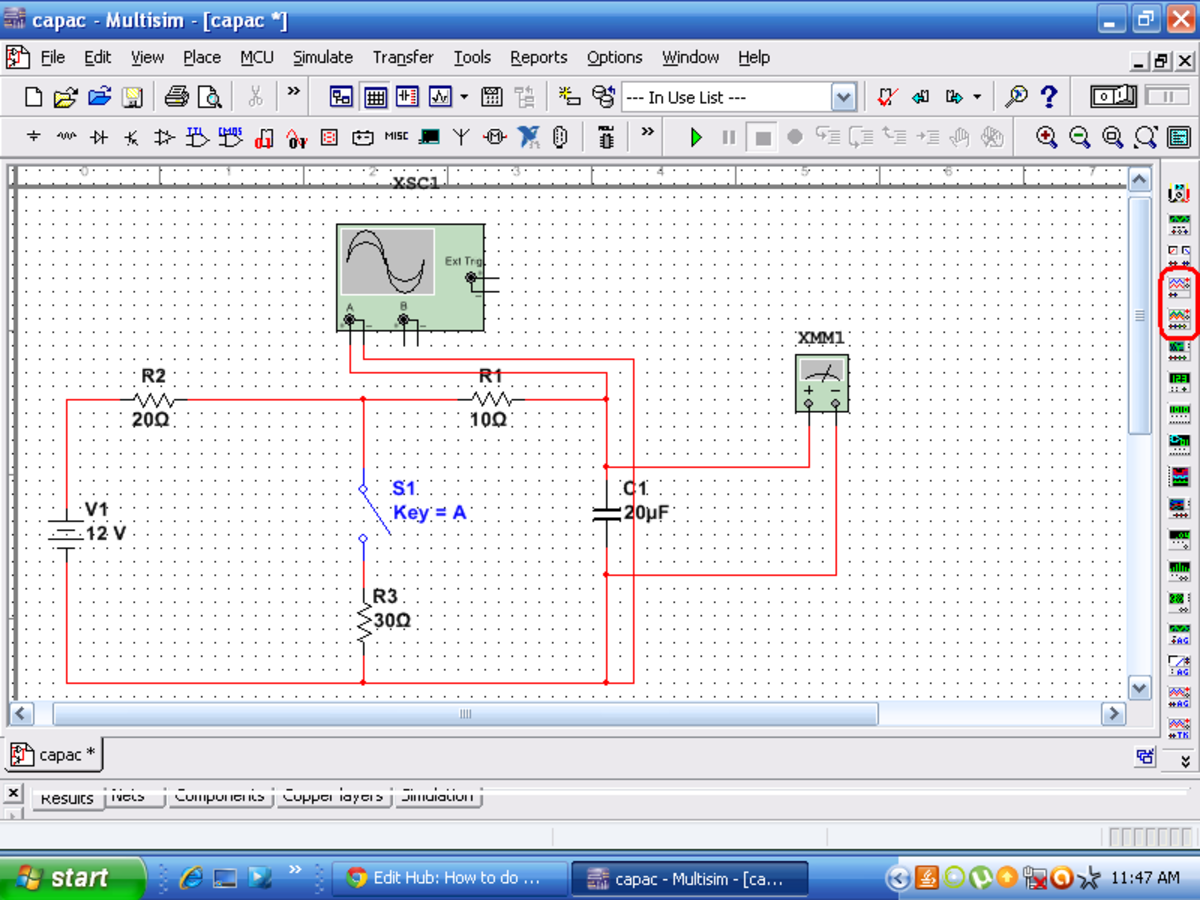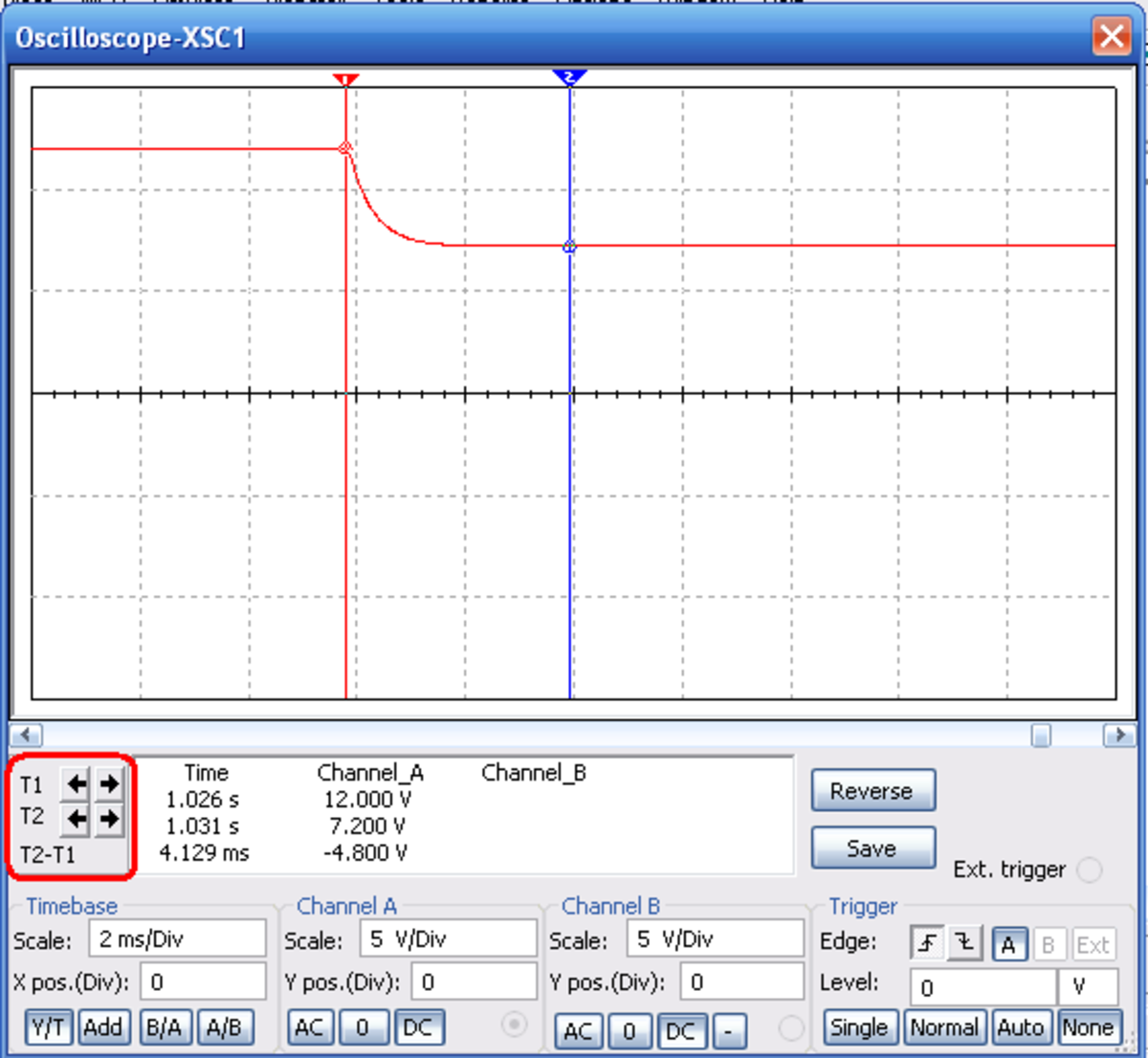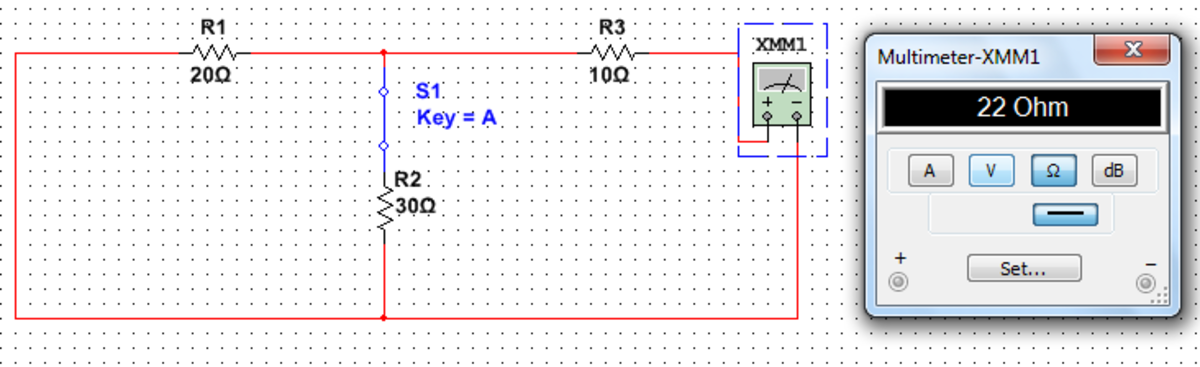Updated date:

# How to do R-C Transient Analysis in Multisim

The Author is a proud Nustian where he studies Electrical Engg. at Pakistan Navy Engineering College, Karachi.This tutorial will teach you how to carry out complete R-C Transient Analysis using Multisim®. It will be very helpful in checking solutions to your textbook questions and will save a lot of time as compared to doing the same analysis by hand. You can even make your own questions and practice them!

Simply follow these steps outlined below to attain the mastery of Multisim transient analysis.

Set up the the circuit as shown below. You will find the oscilloscope in the toolbar towards your right.• Place the oscilloscope such that its two terminals are connected in parallel with the component for which the transient analysis is to be done. For example I have connected it across the capacitor as I intended to find Voltage at time (t) across the capacitor. (+ve and -ve terminals are indicated on the oscilloscope).
• Set the position of the switch (opened or closed) according to your choice and note its key.(A key is a button which will change the position of the switch, in our case its 'A')(here we will examine the circuit with an open switch closing).
• Click on the simulate button to run the circuit.
• Next double click on the oscilloscope (if you see a black screen click on the 'reverse' tab) and you should see a continuously flashing red line, this is the trace for voltage.
• Now to obtain the trace of voltage across capacitor during the transient phase:
1. Click once outside the oscilloscope window.
2. Press the key to close the switch, you should see a change in trace for a small instance.
3. Stop the circuit and scroll back and you should find the trace that looks something like pictured below.Oscilloscope trace for 'Open switch Closing'.
• Adjust the time base and the voltage divisions of the oscilloscope so as to obtain a readable trace.
• Moving the two markers T1 and T2 will give you instantaneous values of the voltage and time as well as their difference (see the red circle).
• Place the two markers as shown in the picture above to find the initial and final values of voltages [ V(0) and V(∞) ].
1. For our analysis V(0) = 12V from marker T1.
2. V( ∞) = 7.2V from marker T2.

Now apply the following equation to find the complete transient response of this RC circuit.

• R is the Thevenin equivalent resistance of the circuit.

## Finding Thevnin equivalent Resistance with Multisim

Two ways to find Thevenin equivalent Resistance:

1. 'Short' the voltage source and 'open' the current source then apply an ohmmeter from where you want the resistance to be measured.
2. Add a voltmeter, note the value, and then add an ammeter to your circuit in place of the voltmeter then divide the value of voltage with current. This will also work with the dependent sources but their must be an independent source in the circuit. (illustrated in the picture below).Finding Thevenin Resistance using an Ohmmeter in Multisim.

To check the results with your textbook just substitute the values and obtain the equation for V(t) and compare it with the answers provided.

To check the result with Multisim move the marker T2 a little backward, substitute the value of time given by T2 - T1 with 't' in the equation given above, solve for V(t) at this value of t. If your answer coincides with the value of voltage given by marker T2 then your answer is correct.

© 2014 StormsHalted